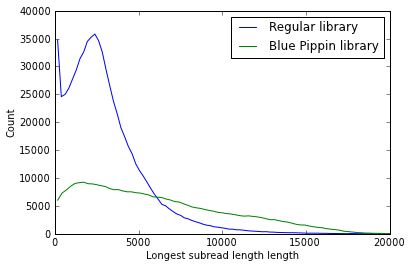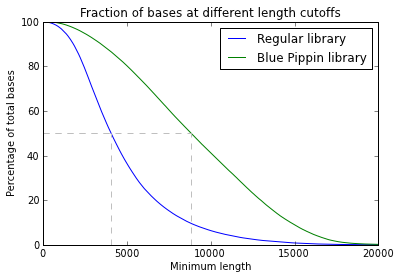Code belonging to the blog post 'Longing for the longest reads: PacBio and BluePippin'

Released under CC BY-SA 2.0

Lex Nederbragt, June 2013

In :
from collections import Counter
from matplotlib import pylab as plt


The following cell sets up the project, and allows for using the notebook on different datasets
Input files are filtered subreads. These are provided by default with software version 2.0 and higher.
For older version, reads need to be filtered and split into subreads.

In :
dataset_name = 'some_name'
dataset_outfolder = 'path/to/some/folder'

# older software versions
files1 = !find /path/to/rundata -name "*filtered_subreads.fasta"
# 2.0 and up
files2 = !find /path/to/rundata -name "*subreads.fasta"

inputs = []
inputs.append(["Dataset name 1", files1])
inputs.append(["Dataset name 2", files2])

In :
def N50(numlist):
"""
Abstract: Returns the N50 value of the passed list of numbers.
Usage: N50(numlist)

Based on the definition from this SEQanswers post

"""
numlist.sort(reverse = True)
s = sum(numlist)
limit = s * 0.5
for l in numlist:
s -= l
if s <= limit:
return l

In :
def load_data(files, max_reads):
"""Parses the subreads fasta file and collects the length of the longest subread for each ZMW ('read of insert')"""
lengths = {}
i = 0
for fname in files:
with open(fname, 'r') as fh:
for line in fh:
line = line.strip().split()
if line.startswith('>'):
i += 1
break
[name, zmw, coord] = line[1:].split('/')
start, stop = coord.split('_')
read_name = name + '_' + zmw
# add length if no entry yet, otherwise use longest length
# 'lengths.get(read_name,0)' returns either the previously recorded length for this read, or 0
return lengths.values()

In :
def rel_cumsum(lengths):
"""Determines the lengths of the reads sorted from short to long,
and the fracttion of the cumualtive sum of reads at that length.
Needed for plotting """
c = Counter(lengths)
total = sum(lengths)
csum = 0
cum_len = []
sort_len = []
for k in sorted(c.iterkeys()):
csum = csum + k * c[k]
cum_len.append((total_len - csum)/float(total_len))
sort_len.append(k)
return sort_len, cum_len


In [ ]:
max_reads =  1E30 #allows for loading a subset by using a smaller number
all_lengths = {}
for indata in inputs:
libname, fnames = indata
print libname
all_lengths[libname] = lengths


Metrics:

In :
N50s = {}
for indata in inputs:
libname, fname = indata
lib_N50 = N50(all_lengths[libname])
N50s[libname] = lib_N50
print "%s\t%i\t%i\t%i\t%i" %(libname,
len(all_lengths[libname]),
sum(all_lengths[libname])/len(all_lengths[libname]),
lib_N50,
max(all_lengths[libname]))

Library	# of reads	Ave. length	Longest subread N50	Longest
Regular library	693275	3009	4041	22298
Blue Pippin library	325407	6045	8820	25931


Plotting length distributions:

In :
num_points = -1 # '-1' for all
for indata in inputs:
libname, fname = indata
y,binEdges=np.histogram(all_lengths[libname][0:num_points],bins=100)
bincenters = 0.5*(binEdges[1:]+binEdges[:-1])
plt.plot(bincenters,y,'-', label = libname)
legend()
plt.xlim(0,20000)
plt.ylabel('Count')
plt.savefig(dataset_outfolder + '/' + dataset_name + '_length_dist.pdf')Plotting fractional distribution:

In :
for indata in inputs:
libname, fname = indata
total_len = sum(all_lengths[libname])
x, y = rel_cumsum(all_lengths[libname])
plt.plot(x, [100*i for i in y], label = libname)
plt.plot([N50s[libname],N50s[libname]],[0,50], ls='dashed', color = '0.75')
plt.plot([0,N50s[libname]],[50,50], ls='dashed', color = '0.75')
legend()
plt.xlim(0,20000)
plt.title('Fraction of bases at different length cutoffs')
plt.xlabel('Minimum length')
plt.ylabel('Percentage of total bases')
plt.savefig(dataset_outfolder + '/' + dataset_name + '_cumul_length_dist.pdf')